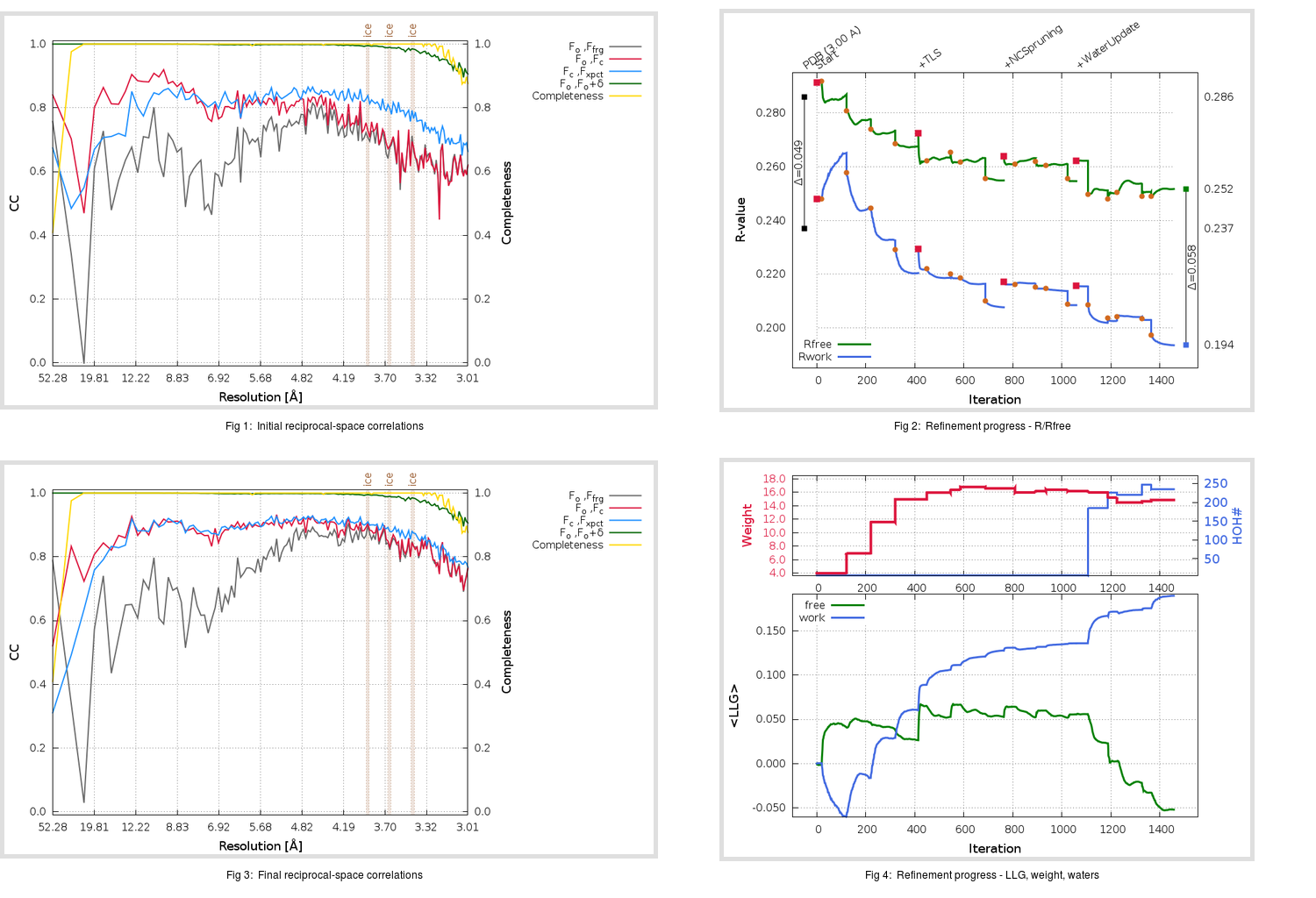Content:

```    Diffraction limits & principal axes of ellipsoid fitted to diffraction cut-off surface:
3.131         1.0000   0.0000   0.0000       a*
2.908         0.0000   1.0000   0.0000       b*
2.910         0.0000   0.0000   1.0000       c*
```

## Deposited

` `
 Date deposited Date data collection Resolution R, Rfree 20071126 20061118 3.00 0.2340 0.2860

Molprobity (CCP4 7.0 version) summary:

```Ramachandran outliers =   0.92 %
favored =  91.95 %
Rotamer outliers      =   3.39 %
C-beta deviations     =     0
Clashscore            =  14.28
RMS(bonds)            =   0.0177
RMS(angles)           =   1.17
MolProbity score      =   2.55
Resolution            =   3.00
R-work                =   0.2340
R-free                =   0.2860
```

```Number of waters      =     6

<B> (all atoms) =   46.99 ( sd =   14.52 ) for       9417 non-hydrogen atoms
<B>   (protein) =   47.01 ( sd =   14.51 ) for       9411 non-hydrogen atoms
<B>     (water) =   24.95 ( sd =    7.43 ) for          6 non-hydrogen atoms
<B>    (others) =    0.00 ( sd =    0.00 ) for          0 non-hydrogen atoms

B min/max       (all non-hydrogen atoms) =   11.62 /   92.83
B min/max   (protein non-hydrogen atoms) =   22.89 /   92.83
B min/max     (water non-hydrogen atoms) =   11.62 /   35.98
B min/max     (other non-hydrogen atoms) =    0.00 /    0.00
```

## BUSTER (re-)refinement

` `

Molprobity (CCP4 7.0 version) summary:

```Ramachandran outliers =   0.17 %
favored =  95.38 %
Rotamer outliers      =   8.18 %
C-beta deviations     =     2
Clashscore            =   5.14
RMS(bonds)            =   0.0125
RMS(angles)           =   1.63
MolProbity score      =   2.29
Resolution            =   3.00
R-work                =   0.1936
R-free                =   0.2517
```

```Number of waters      =   235

<B> (all atoms) =   62.06 ( sd =   20.31 ) for       9646 non-hydrogen atoms
<B>   (protein) =   62.60 ( sd =   20.20 ) for       9411 non-hydrogen atoms
<B>     (water) =   40.25 ( sd =    9.77 ) for        235 non-hydrogen atoms
<B>    (others) =    0.00 ( sd =    0.00 ) for          0 non-hydrogen atoms

B min/max       (all non-hydrogen atoms) =   19.04 /  136.63
B min/max   (protein non-hydrogen atoms) =   31.00 /  136.63
B min/max     (water non-hydrogen atoms) =   19.04 /   77.66
B min/max     (other non-hydrogen atoms) =    0.00 /    0.00
```

Refinement progression:Results:

` `
 File Remark 3BGF_aB_refine.01_04_refine.pdb.gz exact refinement commands are in header 3BGF_aB_refine.01_04_refine.mtz.gz including original deposited data and several re-refinement map coefficients 3BGF_aB_refine.01_04_BUSTER_model.cif.gz including any non-standard compound restraints 3BGF_aB_refine.01_04_BUSTER_refln.cif.gz